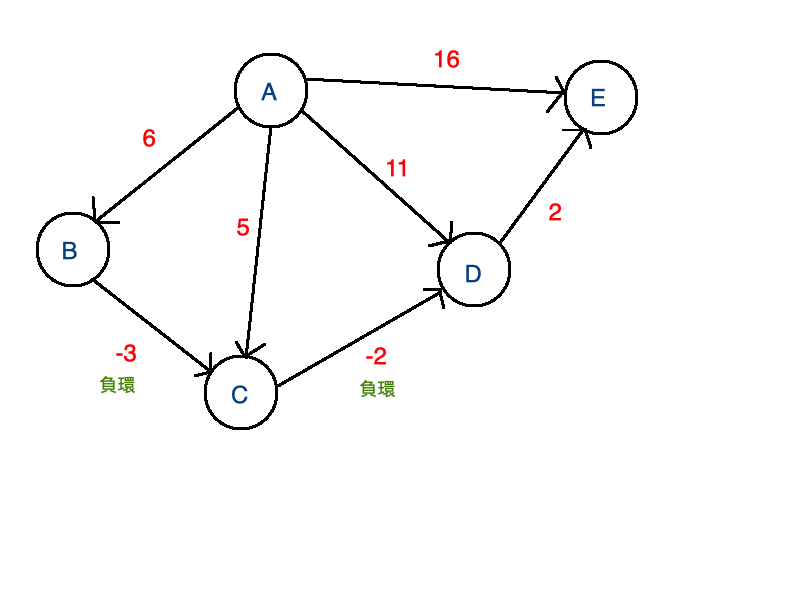DAY 18
0

戴克斯特拉演算法(Dijkstra's algorithm)

Dijkstra's algorithm與Bellman-Ford Algorithm一樣，能夠正確求出有向圖的最短路徑。但圖形中若有負值時，Dijkstra's Algorithm無法求出正確路徑。迴圈中有負環時，並不存在最短路徑。Bellman-Ford Algorithm可以判斷出最短路徑不存在，但Dijkstra's Algorithm會將錯誤的判斷當作正確答案。因此，無法使用Dijkstra's Algorithm無法用於負環的圖形。

from heapq import *
City = {}
G = {}
pq = []
# dis為101個元素的串列，每個元素值都是1000000
dis = *101
# v為101個元素的串列，每個元素值都是0
v = *101
# 將節點名稱轉成數字，使用字串p為輸入參數，將節點名稱p轉換成節點編號
def getCityIndex(p):
# 若p不是City的key，則設定City[p]
if p not in City.keys():
# 對應的值為City的長度
City[p]=len(City)
# 回傳City key的對應值
return City[p]

class Edge:
def __init__(self, s, t, w):
# s：邊的起點，t：邊的終點，w：邊的權重
self.s = s
self.t = t
self.w = w

n = int(input())
for i in range(m):
# 每次輸入三個資料，表示邊的兩個頂點到變數a與b，邊的權重到變數w
a, b, w = input().split()
a = getCityIndex(a)
b = getCityIndex(b)
w = int(w)
e1 = Edge(a, b, w)
e2 = Edge(b, a, w)
if a in G.keys():
G[a].append(e1)
else:
G[a]=[e1]
if b in G.keys():
G[b].append(e2)
else:
G[b]=[e2]

# 輸入起點節點名稱，傳入getCityIndex轉換成節點編號，變數s參考到此節點編號
s = getCityIndex(input())
# 建立有兩個元素的tuple，目標點起始點為s
p = (0, s) #(距離,目標點)
heappush(pq, p)
# 表示出發點的最短距離為0
dis[s]=0

while len(pq) > 0:
# 使用heappop取出最上面元素（起始點到此點是最短距離）
p = heappop(pq)
# s為p的第二個元素，為該邊的目標點起點，是下一個起點
s = p
# 若v[s]=0，設定v[s] = 1，表示起始點到節點編號s是最短路徑
if v[s] == 0:
v[s] = 1
for edge in G[s]:
if v[edge.t] == 0:
if dis[edge.t] > dis[s] + edge.w:
dis[edge.t] = dis[s] + edge.w
p = (dis[edge.t], edge.t) #(距離,目標點)
heappush(pq, p)

for i in range(len(City)):
print(dis[i]," ", sep="",end="")
print()

負環